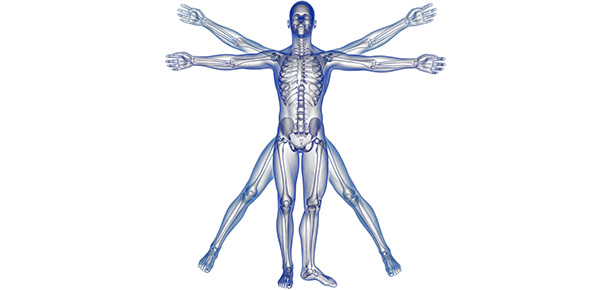# Ammar Physiology Exam

30 Questions | Total Attempts: 762Settings.

Related Topics
• 1.
The last human instinct activated during the life of human is :
• A.

Maternity

• B.

Food

• C.

Sex

• D.

• 2.
Which of the following systems is NOT directly involved in maintaining homeostasis?
• A.

Renal system.

• B.

Immune system.

• C.

Circulatory system.

• D.

Respiratory system.

• 3.
Sweating initiated in responses to a rise in body temperature that occurs on exposure to a hot environment, evaporation of the sweat cools the body. If environmental temperature rise 17 cc and the gain is 33, so body temperature will be:
• A.

Normal +0.5.

• B.

Normal +1

• C.

Normal -1

• D.

Normal -0.5.

• 4.
If two control systems, have gain of 4 and 3 respectively then which of the following is correct:
• A.

First system correction is better than the second system.

• B.

Second system correction is better than first system correction

• C.

First system error is higher than second system error

• D.

None of the above

• 5.
The rate of O2 diffusion across a cell membrane could be increased by increasing the:
• A.

Concentration gradient (difference in concentration) across the membrane.

• B.

Plasma membrane thickness.

• C.

Amount of ATP.

• D.

Number of carrier proteins in the plasma membrane.

• 6.
Facilitated diffusion:
• A.

Saturate at high solute concentration.

• B.

Active diffusion.

• C.

Rate of diffusion is dependent on concentration gradient only.

• D.

Na+/K+ pump is an example.

• 7.
Blood analysis showed that a hematocrit is 40٪ assume that the plasma volume is 3000 the blood volume of the patient is in liters is :
• A.

3

• B.

6

• C.

5

• D.

5.5

• 8.
All of the following creatures have maternity instinct except :
• A.

Humans

• B.

Birds

• C.

Pigs

• D.

Turtles

• 9.
The molarity of a 4% solution of NaCI is 680 mmol/L. The molecular weight of NaCI is 58.5. What is the osmolarity of a 2% solution of NaCI (in mOsm/L)?
• A.

680

• B.

340

• C.

1360

• D.

300

• 10.
A patient weighs 50 kg, and has a fluid distribution and osmolarity that are normal (i.e. total body fluid is 60 % of body weight, & ICS is 40% of body weight, total osmotic content is 9000 mosmolar). This individual's osmolarity of body fluid would be:
• A.

250

• B.

600

• C.

150

• D.

300

• 11.
Cell volume:
• A.

Will decrease when active Na-K transport is inhibited ￼ ￼

• B.

Will increase when active Na-K transport is inhibited ￼ ￼

• C.

Nothing will happen when Na-K transport is inhibited ￼

• D.

Non of the above

• 12.
A man drinks 2L of iso-tonic fluid these two liters will cause:
• A.

Decrease in ECF volume

• B.

Increase in ECF volume

• C.

Increase in ICF volume

• D.

Decrease in ICF volume

• 13.
Extrusion of big materials to the exterior of the cell through the plasma membrane is called:
• A.

Exocytosis

• B.

Endocytosis

• C.

Pinocytosis

• D.

All of the above

• 14.
When you measured the efficiency of Negative feedback of one of the control systems, you got that the control system corrects 90% of the changes, that means the gain equals:
• A.

10/1

• B.

20/2

• C.

27/3

• D.

• 15.
If there is an increase in the neutrophils , this means the body has:
• A.

Bacterial infection

• B.

Parasitic infection

• C.

Allergy

• D.

All of the above

• 16.
The life span of RBCs is:
• A.

120 days

• B.

Hours

• C.

120 hours

• D.

80 days

• 17.
Which of the following statements is incorrect :
• A.

Vitamin B12 deficiency will lead to megaloblastic anemia.

• B.

Folic acid deficiency will lead to megaloblastic anaemia

• C.

Iron deficiency will lead to macrocytic anemia

• D.

All are correct

• 18.
All of the following proteins are produced in the liver except :
• A.

Albumin

• B.

Fibrinogen

• C.

Alpha globulin

• D.

Gamma globulin

• 19.
Serum is :
• A.

Normal plasma.

• B.

Plasma with low O2 pressure

• C.

Plasma with low fibrinogen and coagulation factors concentration.

• D.

Plasma with low albumin.

• 20.
When k+ channels open............ Happens:
• A.

Action potential

• B.

Depolarization

• C.

Repolarization

• D.

Resting membrane potential

• 21.
If membrane potential changed from +30 Mv to -120 Mv (resting membrane potential = -90 Mv) we will have:
• A.

Repolarization

• B.

Depolarization

• C.

Action potential

• D.

Hyperpolrization

• 22.
1. Which of the following ions will increase inside the skeletal muscles during contraction:
• A.

Na+

• B.

K+

• C.

Ca+2

• D.

Cl-

• 23.
Which of the following neurotransmitter will be released at neuromuscular junction:
• A.

Acetylcholine

• B.

Glutamate

• C.

• D.

• 24.
Which of the following is not a step in the neuromuscular transmission :
• A.

Calcium ions influx to the Presynaptic neuron.

• B.

Neurotransmitter release to the synaptic cleft.

• C.

Increase Na-K pump activity.

• D.

Release of calcium ions from sarcoplasmic reticulum to sarcoplasm.

• 25.
When a stimulate is applied to a nerve cell it :
• A.

Open sodium voltage channels.

• B.

Activates calcium inward current .

• C.

Change the membrane permeability to ions.

• D.

Opens chloride voltage influx.